## Estimate Frequency Response with Linearization-Based Input Using Model Linearizer

When you have a linear model representing the portion of your Simulink® model that you want to estimate, you can use that model to generate the input signal. This alternative to manually specifying the estimation signal (as shown in Estimate Frequency Response Using Model Linearizer) can be useful when you are using frequency response estimation to validate a model obtained through linearization. This example shows how to perform frequency response estimation in Model Linearizer using an input signal that is based on the dynamics of an exact linearization of the model.

In this example, linearize a Simulink model to obtain the linear model you use to generate the estimation input signal.

```sys = 'scdDCMotor'; open_system(sys)```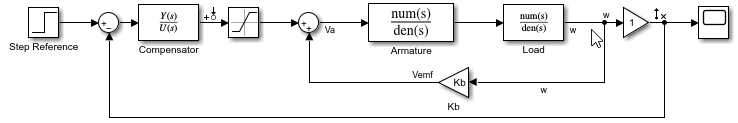This model contains predefined linear analysis points. There is an input point at the compensator output and an open-loop output after the unit gain block. The response from the defined input to the defined output is the response of the inner loop of the model, with the outer loop open.

2. In the Simulink model window, in the Apps gallery, click Model Linearizer.

3. Linearize the model using the predefined analysis points and using the model initial conditions as the operating point.

On the Linear Analysis tab, clickBode.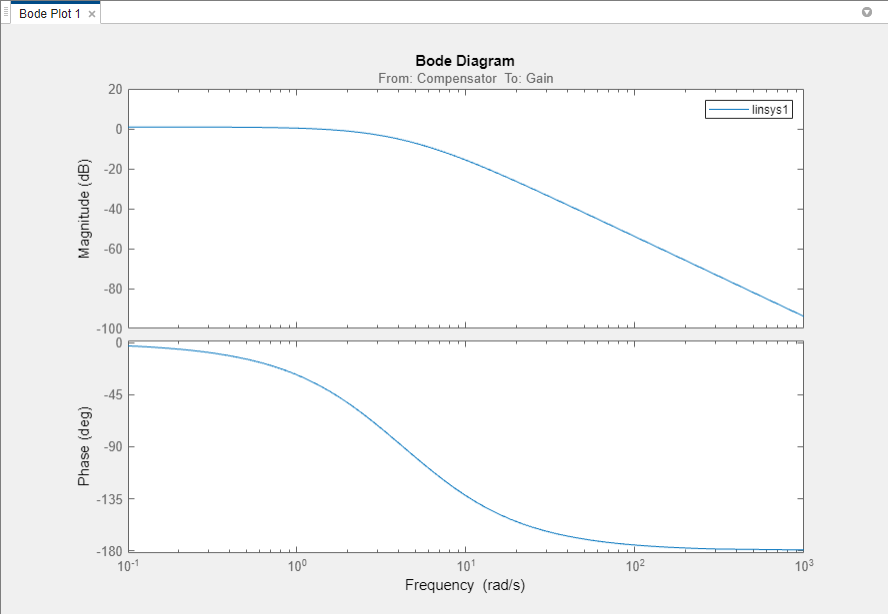A new linearized model, `linsys1`, appears in the Linear Analysis Workspace.

### Create Sinestream Input Signal

1. On the Estimation tab, in the Input Signal drop-down list, select `Sinestream`.

2. Initialize the input signal frequencies and parameters based on `linsys1`.

In the Create sinestream input dialog box, click .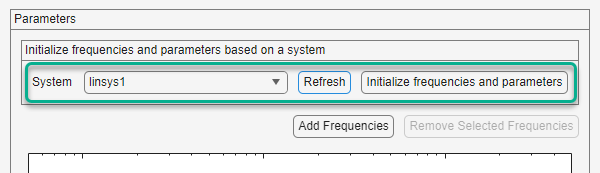The frequency content viewer is populated with frequency points. The software chooses the frequencies and input signal parameters automatically based on the dynamics of `linsys1`. The software also automatically initializes other parameters of the sinestream signal, including:

• Amplitude

• Number of periods

• Settling periods

• Ramp periods

• Number of samples at each period

3. Select all the frequency points.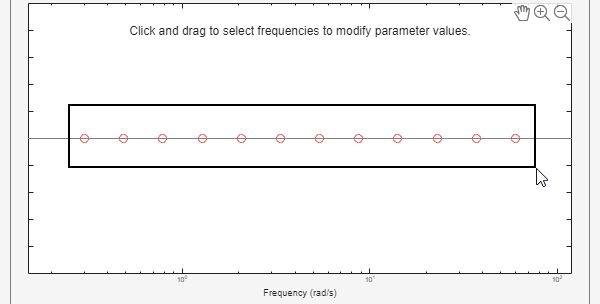4. Specify the amplitude of the input signal.

Enter `1` in the Amplitude box.

5. Create the input sinestream signal.

Click . The input signal `in_sine1` appears in the Linear Analysis Workspace.

### Estimate Frequency Response

ClickBode Plot 1 to estimate the frequency response.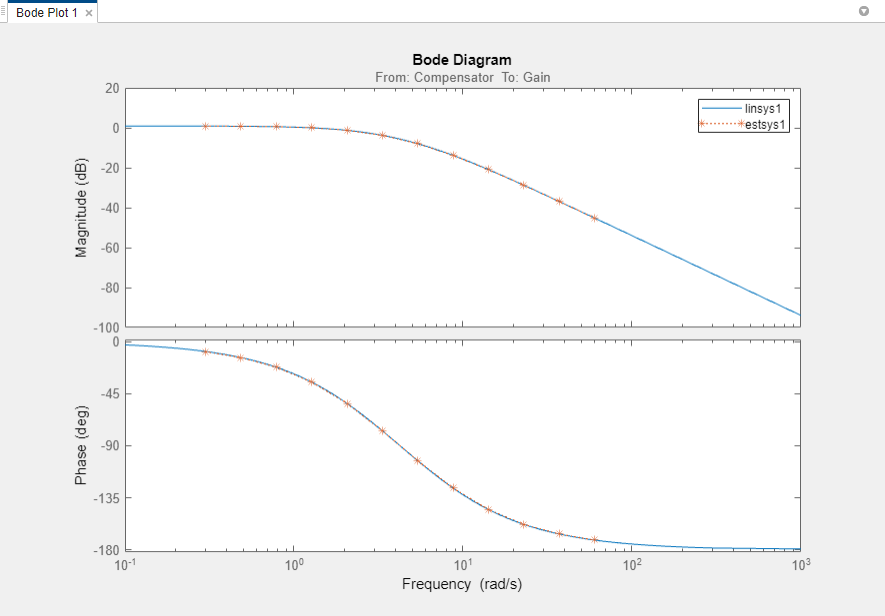The estimated system, `estsys1`, appears in the Linear Analysis Workspace and the its frequency response is added to Bode Plot 1.

The frequency response for the estimated model matches that of the linearized model. You can use this approach to validate an exact linearization.

## Related Topics